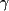Home# Raman spectroscopy of hcp-Fe to 152 GPa

 We measured the Raman spectra of hcp-Fe from 15 to 152 GPa using the diamond anvil cell and ultrapure synthetic diamond anvils. These results were published in Science in june 2000. The hcp phase of iron has one Raman active mode of E2g symmetry. It exhibits a sublinear frequency increase with pressure. The first figure shows our measurements (blue circles and green squares) and predictions from first-principles calculations for ambient temperature (in red). The pressure dependence of the Raman mode can be used to calculate the Grüneisen parameter of the raman mode, and then approximate the thermal Grüneisen parameter and its volume dependence. Fitting the relationwhere0 is the extrapolated value at zero pressure and q the volume depence, we find0 = 1.68 (20) and q = 0.7 (5). In the hcp-lattice, the E2g Raman mode and the C44 elastic modulus are properties of the same phonon branch. Using phenomenological modeling, they can be related bywhere M is the atomic mass of iron, c and a unit cell parameters, andthe frequency of the optical phonon. The C44 we deduce is compared with results from first principles calculations and lattice strains experiments in the second figure. These results are of great importance for understanding the high-pressure thermodynamic and elastic properties of hcp-Fe, the major constituent of the Earth's inner core. It also proves the feasability of Raman measurements on metals under extreme pressure conditions. For more informations and references: Merkel S., A.F. Goncharov, H.K. Mao, P. Gillet and R.J. Hemley, Raman spectroscopy of Iron to 152 Gigapascals: Implications for Earth's Inner Core, Science, Vol. 288, pp. 1626-1629, 2000 The data from the study. Figures
Sebastien Merkel, 06/2001

## © Sébastien Merkel, Université de Lille, France

Home > FeRaman > index.php